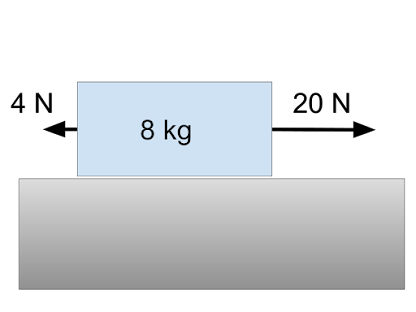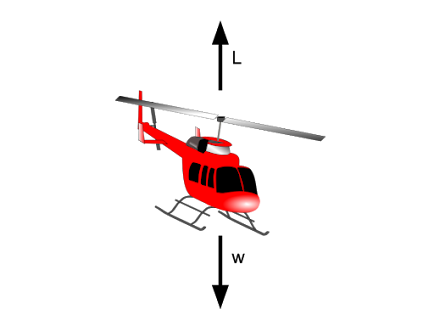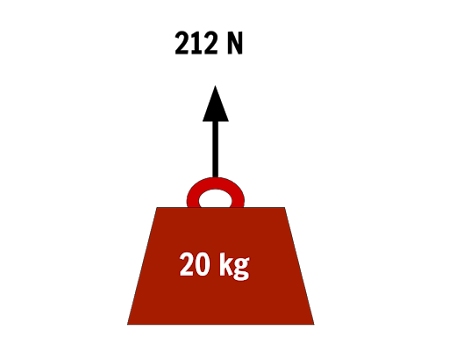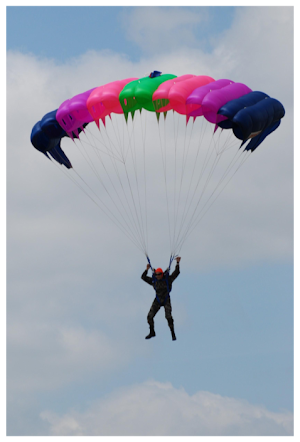Unbalanced Forces Quiz 01
This quiz tests your mastery of drawing force diagrams and finding unbalanced forces.
A block of mass 8 kg is pulled along a table with a constant force of 20 N. There is a frictional force of 4 N between the block and the table. If the block starts from rest its speed after 2 s is
1 pointA helicopter of mass 8,000 kg accelerates upwards at 1.0 m/s2. The magnitude of the lift force from the helicopter`s rotor is
1 pointThe graph below shows the velocity time graph of a trolley of mass 2 kg being pulled with a forward force of 20 N. The backward force F must be
1 pointA 20 kg block is pulled upwards with a constant force of 212 N. Assuming the acceleration due to gravity is 9.8 m/s2 then the acceleration of the block is
1 pointA parachutist of mass 80 kg is accelerating downwards at 0.4 m/s2. If the acceleration due to gravity is 9.8 m/s2 then the upward force acting on the parachutist is
1 point